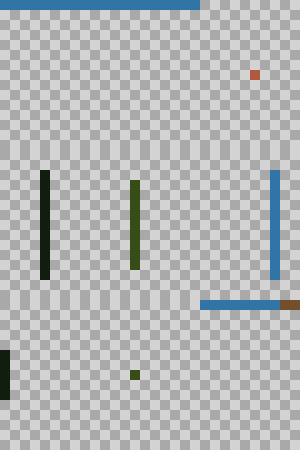## Hungry Cat Picross solver

This program is an attempt to automatically solve a Hungry Cat Picross grid with a simple algorithm.

The principle of the game is simple, yet complex to solve: each pixel has different possible values. It is not possible to test all combinations, so we have to be smarter.

## Imports

``````import os
import math
import re
import numpy as np
import matplotlib.colors
import PIL
import copy
from PIL import Image, ImageDraw, ImageFont
``````

## Utility functions

`arraytopix` allows to transform easily a numpy array to a PIL image

`colorto2rgb` transforms a color name to a RGB value

``````def arraytopix(array, palette, pixsize=1):
npi = np.zeros((array.shape, array.shape, 3), dtype="uint8")
for i in range(npi.shape):
for j in range(npi.shape):
npi[i,j] = palette[array[j,i]]
pal = Image.fromarray(npi).resize((array.shape*pixsize, array.shape*pixsize), PIL.Image.NEAREST)
return pal
``````
``````def colortorgb(color):
return tuple(int(x*255) for x in matplotlib.colors.to_rgb(color))
c2rgb=colortorgb
def gethue(x):
return matplotlib.colors.rgb_to_hsv(matplotlib.colors.to_rgb(x))
def getsat(x):
return matplotlib.colors.rgb_to_hsv(matplotlib.colors.to_rgb(x))
def getbri(x):
return matplotlib.colors.rgb_to_hsv(matplotlib.colors.to_rgb(x))
``````

## Color names

As we may want to type ourselves the problems in a first step, let’s define some color names (from matplotlib colors)

``````cw, ch = 900, 680
bw, bh = 30, 25
sx, sy = 157, 27
colorpic = Image.new('RGB', (cw,ch), (255,255,255))
fnt = ImageFont.truetype('arial.ttf', 13)
draw = ImageDraw.Draw(colorpic)

colors = [x for x in matplotlib.colors.get_named_colors_mapping().keys() if ":" not in x and len(x) > 1]
satura = [x for x in colors if getsat(x) > 0.05]
grays  = [x for x in colors if getsat(x) <= 0.05]
colors = sorted(grays, key=getbri) + sorted(satura, key=gethue)
x = 0
y = 0
for color in colors:
draw.rectangle([x,y,x+bw,y+bh], fill=c2rgb(color), outline=(0,0,0))
draw.text((x+bw+5,y+4), color, font=fnt, fill=(10,10,10))
y+=sy
if y > ch - bh:
y = 0
x += sx
colorpic
``````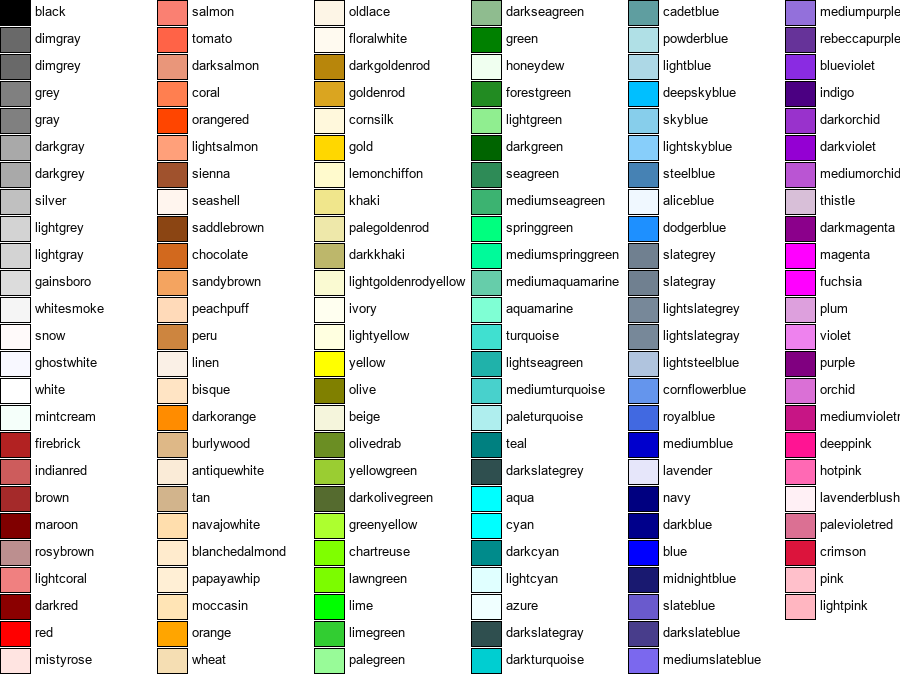## Testing the utility function to generate an empty grid (starting point)

``````a=np.fromfunction(lambda i,j: (i+j)%2, (10,15), dtype="uint8")
pal=[c2rgb("lightgray"),c2rgb("darkgray")]
arraytopix(a,pal,20)
``````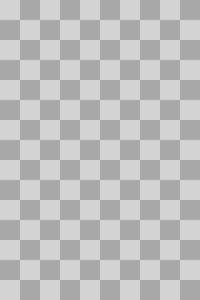## General Algorithm

Let’s get to the meat of the algorithm!

### Definitions

We will naturally name the different structures of the problem.

• Columns, rows: corresponds to the columns and rows definitions of the problem. We do not name the actual columns and rows of the picture unless we are working with the pixel for image-related functions.
• Lines: Either a column or a row. A line is what allows us to make decisions. We never make decisions with using more than one line at a time.
• Matrix: The matrix of all possibilities of colors. This matrix can be stored flat or as an actual matrix.
• Colors: Available colors. Usually there are less than 4 colors.

## Algorithm

First, we have to realize that columns and rows are completely identical and independant. The only way information “propagates” is from the matrix of pixel itself. We do not touch at all the numbers of “non runs pixels” and “runs pixels”. “Runs” pixel are a contiguous group of cells/pixels without holes in them. “Non runs” pixels are non contiguous group with hole with them, or are a group pixel of 1 (this is not distinguished within the game).

Thus, what we do for each column is also done for each row.

1. For each list, we count the number of remaining color for each color. A color is set if it’s the only choice for a pixel. If numcolors - numfixedcolors == 0, then no other pixels can be this color. We do a pass where we remove all the colors from the other pixels. We also count the number of possible location for each color. If numcolors == numlocations, then all those locations are this color. We mark those.
2. For each location, if the pixel would create a run at that location, then we mark this location as impossible for this color. Non runs means that there is at least one hole.
3. For each run, we try all possible position. A position is possible if and only if all its cell still have the color as possible, and no other cell has the color as fixed. If for all run a cell is valid, then it’s marked as the color. If for all run a cell is invalid, then it’s marked as not this color.

Repeat until there are only fixed cells.

## Caveats

This algorithm does not follow the games where you have to “guess” or “try” first. Take the following example (?? means unconstrained):

/????????????
④R 2BRBRBR_R_RBRB

For each undecided cell (RB), both a run of R is possible. However, if we “try” to put the run on the left or right (not center), then the blues are creating a run. But it cannot be proven in advance.

For this problem, we solve with the following approach: On a general sense, the grid has “noise” or a certain amount of information. With time, this amount should only increase (we have more and more info). We can see that actually, one value is always decreasing: the sum of the number of possible color for each pixel. If that value doesn’t decrease anymore, then we have to make a guess.

## Guess

We take all the possible pixels and guess at random (starting with first pixel). We then fix that pixel and try to resolve. Please note that introducing “guesses” forces also the code to be able to validate the matrix: after all, a guess could be wrong (whereas in the previous steps we can only make valid moves, hence never invalidating the grid).

We are trying all the “non fixed” pixels, in order, starting with first color. While it works in practice, it would be much better to select first:

• Cells with only two choices;
• Cells where the fixing immediately introduces chain reaction (i.e., not a “useless” guess). However, since after the first steps the matrix is already quite constrained, in practice in the few first pixels this chain reaction occurs and the move is either valid or invalid. We simulate a human by not looking at more than 3 steps ahead. If we can’t deduce anything, then it’s probably not the good pixel to begin with.

If a guess is invalid, we have to roll back to previous state. We do this by using a “deepcopy” mechanism that clones ourselves before starting a recursion. It is heavy handed, as actually we would only need to duplicate the inner matrix. If we were solving very large puzzles, we could even not duplicate ourselves, and instead “undo” each step of the recursion if the solving fails.

If a guess is valid, then the solution of that half-filled grid is the end of our current solution. We combine them and return the solution. This approach works well and even allows for the recording of the solution.

## Animation

Since we can record each step of constraining, we can record an “animation” of the solution. Right now, we record each time the information value increases (the noise value decreases). We could have a (maybe ?) nicer rendering by recording each pixel as it becomes fixed. This is left as a exercise for the reader.

## Bonus

The algorithm is also slow. We can speed it up by “freezing” columns or rows (i.e., lines) which have all their color found.

``````""" This is the main holder of constraint.
A line is a group of non runs, runs (mutually exclusive for each color) and cells.
A line by itself can check constraints, however we do it in the main class for convinience.
"""
class picxline():

def __init__(self, size, name=""):
self.size  = size
self.name  = name
self.nruns = [ 0 ] * size
self.runs  = [ 0 ] * size
self.mixed = [ 0 ] * size
self.frozen = False

def parse(self, colors, colordict):
while colors:
m = re.match("([A-Z])+([0-9]+)", colors)
if m:
index = colordict[m.group(1)]
number = m.group(2)
self.nruns[index] = int(number)
m = re.match("([a-z])+([0-9]+)", colors)
if m:
index = colordict[m.group(1).upper()]
number = m.group(2)
self.runs[index] = int(number)
colors=colors[1+len(number):]
self.compute_mixed()

def parsexml(self, colors):
data = [int(x) for x in colors.text.split(",")[:-1]] #removes the last empty cell
assert len(data) == self.size, "Not the good amount of colors"
for i, c in enumerate(data):
if c > 100:
self.runs[i] = c-100
else:
self.nruns[i] = c
self.compute_mixed()

def compute_mixed(self):
for i in range(self.size):
assert (self.nruns[i] == 0) or (self.runs[i] == 0), "There are two pixels at the same place {} - {}".format(self.nruns, self.runs)
self.mixed[i] = self.nruns[i] + self.runs[i]

def set_line_data(self, data):
self.data = data

def freeze(self):
self.frozen = True

def is_frozen(self):
return self.frozen

def __repr__(self):
return "{} - nruns: {}, runs:{}, mixed:{}, data:{}".format(self.name, self.nruns, self.runs, self.mixed, self.data)
``````

## picxproblem

Here we define a class that is able to load, solve and display a grid. Roughly, the class is divided in 3 parts: a loading part (two formats supported), a solving part (from `remove_pixel_color` to `solve`) and a display part. We abuse the fact that in python everything is a reference to build the various lines. Please note that at no point, for the solving algorithm, we need to have an orthogonal grid: we could have triangular grid, hexagonal, a grid with holes… The solving algorithm would work (loading&displaying wouldn’t of course).

The format is as follow:

• Color definition: LETTER1:NAME1 LETTER2:NAME2 etc… For example, R:red B:blue
• Column&row definition: Uppercase means non-run, lowercase means run, followed by the number of occurences. For example, r2B3 b5 R4B1

The xml format is not discussed here.

### Solving

Apart from the algorithm described above, we only have helper functions to count the runs, the colors, etc.

### Displaying

We have two way of displaying: creating a picture or creating an HTML table. The HTML table is less “pretty” but allows for displaying nicely inside the notebook itself.

``````class picxproblem():

def __init__(self, width = 0, height = 0, colors = "", columns = None, rows = None, xml_filename=None):
self.backgroundpal = [c2rgb('darkgray'), c2rgb('lightgray')]
if xml_filename:
self.parsexml(xml_filename)
else:
self.width = width
self.height = height
colors = {x.split(":"):c2rgb(x.split(":")) for x in colors.split(" ")}
self.palette = [colors[x] for x in sorted(colors.keys())] + self.backgroundpal
self.numcolors = len(colors)
self.initlines()
self.colortonum = {}
for i,c in enumerate(sorted(colors.keys())):
self.colortonum[c.upper()]=i
if columns:
self.parse(columns, self.x_lines)
if rows:
self.parse(rows, self.y_lines)

self.initmatrix()
for i, x in enumerate(self.x_lines):
x.set_line_data(self.get_xaxis(i))
for i, x in enumerate(self.y_lines):
x.set_line_data(self.get_yaxis(i))
self.lines=self.x_lines+self.y_lines

def initlines(self):
self.x_lines = [ picxline(self.numcolors, "col{}".format(i)) for i in range(self.width) ]
self.y_lines = [ picxline(self.numcolors, "row{}".format(i)) for i in range(self.height)]

def initmatrix(self):
self.matrix  = [ () ] * self.width * self.height
for i in range(self.width * self.height):
self.matrix[i] = list(range(self.numcolors))

def get_pixel(self, x, y):
return self.matrix[x + y*self.width]

def get_xaxis(self, x):
ret = [ [] ] * self.height
for y in range(self.height):
ret[y] = self.get_pixel(x,y)
return ret

def get_yaxis(self, y):
ret = [ [] ] * self.width
for x in range(self.width):
ret[x] = self.get_pixel(x,y)
return ret

def parse(self, data, lines):
data = data.split(" ")
for i, line  in enumerate(data):
lines[i].parse(line, self.colortonum)

def parsexml(self, xml_filename):
from lxml import objectify
if xml_filename.startswith("http://") or xml_filename.startswith("https://"):
import requests
root = objectify.fromstring(requests.get(xml_filename).text)
else:
root = objectify.parse(open(xml_filename,"r")).getroot()
rows=root.line[:]
columns=root.column[:]
colors=root.colors[:]
self.width=len(columns)
self.height=len(rows)
self.numcolors=len(colors)
self.initlines()
self.palette = []
for color in colors:
self.palette.append( (int(float(color.get("r"))*255),
int(float(color.get("g"))*255),
int(float(color.get("b"))*255)) )
self.palette += self.backgroundpal
for i, column in enumerate(columns):
self.x_lines[i].parsexml(column)
for i, row in enumerate(rows):
self.y_lines[i].parsexml(row)

def remove_pixel_color(self, x, color):
if len(x) > 1 and color in x:
x.remove(color)

def set_pixel_color(self, x, color):
if color in x:
for j in range(self.numcolors):
if j != color and j in x:
x.remove(j)

def remove_color(self, _list, color):
for x in _list:
self.remove_pixel_color(x, color)

def set_color(self, _list, color):
for x in _list:
self.set_pixel_color(x, color)

def count_colors(self, _list, color):
possible = [x if color in x else [] for x in _list]
fixed = [1 if len(x) == 1 else 0 for x in possible]
return sum(fixed), len(possible) #number of fixed colors and number of possible colors

def filter_by_color(self, _list, color):
possible = [True if color in x else False for x in _list]
fixed    = [True if color in x and len(x) == 1 else False for x in _list]
return possible, fixed

def get_longest_run(self, _list):
current = 0
longest = 0
for x in _list:
if x:
current += 1
else:
current = 0
longest = max(longest, current)
return longest

# Here we do the first steps of the algorithm
def solve_step1(self, _list, colors):
for color, colorcount in enumerate(colors):
nfixed, npossible = self.count_colors(_list, color)
if nfixed == colorcount:
self.remove_color(_list, color)
if npossible == colorcount:
self.set_color(_list, color)

#Second step
def solve_step2(self, _list, colors):
for color, colorcount in enumerate(colors):
possible, fixed = self.filter_by_color(_list, color)
for i, x in enumerate(_list):
if possible[i]:
new_fixed = fixed[:] #make a copy
new_fixed[i] = True
if self.get_longest_run(new_fixed) >= colorcount and colorcount > 1: #forbidden
self.remove_pixel_color(x, color)

def checkposition(self, position, length, _list, color):
for i, c in enumerate(_list):
if (i < position or i >= position+length):
if color in c and len(c) == 1:
return False
else:
if color not in c:
return False
return True

#Third step
def solve_step3(self, line, colors):
size = len(line)
for color, colorcount in enumerate(colors):
if colorcount > 1: #a run should be > 1
always_outside = [True] * size
always_inside  = [True] * size
for i in range(0, size - colorcount + 1):
check = self.checkposition(i, colorcount, line, color)
if check:
for j in range(size):
if j<i or j > i + colorcount - 1:
always_inside[j] = False
else:
always_outside[j] = False
for i in range(size):
if always_inside[i]:
self.set_pixel_color(line[i], color)
if always_outside[i]:
self.remove_pixel_color(line[i], color)

def get_runs_and_nruns(self, data, color):
nrun = 0
run  = 0
crun = 0
for cell in data:
if len(cell) == 1 and cell == color:
nrun += 1
crun += 1
run = max(run, crun)
else:
crun = 0
return run, nrun

def check_constraints(self, line):
data = line.data
for color in range(self.numcolors):
run, nrun = self.get_runs_and_nruns(data, color)
#print(data, color, run, nrun)
if ((line.runs[color] > 0 and line.runs[color] < run) or
(line.runs[color] > 0 and nrun > run) or
(line.nruns[color] > 0 and line.nruns[color] < run) or  #We have two seemingly similar conditions here because a "non run" and a "run" of 1 are the same thing.
(line.nruns[color] > 1 and line.nruns[color] <= run) or #So it is allowed to have nrun == 1 && computed_run ==1. Other solution is to force run==1 -> run=0
(line.mixed[color] < nrun)):
return False
return True

def get_information_value(self):
return sum([len(x) for x in self.matrix]), sum([1 if len(x) == 1 else 0 for x in self.matrix])

def solve(self, showprogress=False, recordprogress=False, check_constraints=False, recursion_level = 0, pixel_size=30):
if recursion_level == 3: #We limit the recursion as it we are already trying 3 pixel in advance, it's probably not the good part to look at
return False
self.solution_animation = []
while True:
before_infor, before_fixed = self.get_information_value()
for line in self.lines:
self.solve_step1(line.data, line.mixed)
self.solve_step2(line.data, line.nruns)
self.solve_step3(line.data, line.runs)
_, after_fixed = self.get_information_value()
if recordprogress and after_fixed > before_fixed:
self.solution_animation.append(self.topix(pixel_size=pixel_size))
before_fixed = after_fixed

if check_constraints:
for line in self.lines:
if not self.check_constraints(line):
#print("Constraint violated at line: {}".format(line))
return False
after_infor, after_fixed = self.get_information_value()
if showprogress:
display(self.topix(pixel_size=pixel_size))
if after_fixed == len(self.matrix):
return True
if after_infor == before_infor:
for i, cell in enumerate(self.matrix):
if len(cell) == 2:
backup_cell = cell[:]
for c in range(len(backup_cell)):
new = copy.deepcopy(self)
#print("Trying color #{} at cell {}-{} : {}".format(c, i%self.width, i//self.width, cell))
new.set_pixel_color(new.matrix[i], backup_cell[c]) #we fix one color
if new.solve(check_constraints = True, recursion_level = recursion_level + 1, recordprogress = recordprogress, showprogress = showprogress, pixel_size=pixel_size):
self.matrix = new.matrix
self.solution_animation += new.solution_animation
return True
return False
return True

def topix(self, pixel_size=30):
simplified = np.zeros((self.width, self.height), dtype="uint8")
for i in range(self.width):
for j in range(self.height):
pixel = self.get_pixel(i,j)
if len(pixel) == 1:
simplified[i,j] = pixel
else:
simplified[i,j] = (i+j)%2 + self.numcolors
return arraytopix(simplified, self.palette, pixel_size)

def _repr_html_(self):
circled =  "⓪①②③④⑤⑥⑦⑧⑨⑩⑪⑫⑬⑭⑮⑯⑰⑱⑲⑳"
lines = []
for color in range(self.numcolors):
line = '<td style="height:32px;width: 32px">&nbsp;</td>'*self.numcolors
for column in range(self.width):
line += '<td style="height:32px;width: 32px;color:rgb{}; text-align:center; {}">'.format(self.palette[color], shadow_css)
if self.x_lines[column].runs[color] > 0:
line += circled[self.x_lines[column].runs[color]]
elif self.x_lines[column].nruns[color] > 0:
line += str(self.x_lines[column].nruns[color])
line += "</td>"
lines.append(line)
for row in range(self.height):
line = ""
for color in range(self.numcolors):
line += '<td style="height:32px;width: 32px;color:rgb{}; text-align:center; {}">'.format(self.palette[color], shadow_css)
if self.y_lines[row].runs[color] > 0:
line += circled[self.y_lines[row].runs[color]]
elif self.y_lines[row].nruns[color] > 0:
line += str(self.y_lines[row].nruns[color])
line += "</td>"
for i in range(self.width):
line += '<td style="height:32px;width: 32px'
pixel = self.get_pixel(i,row)
if len(pixel) == 1:
line += '; background-color:rgb{}">'.format(self.palette[pixel])
else:
line += '">&nbsp;'
line += '</td>'
lines.append(line)
return '<table><tr>'+'</tr><tr>'.join(lines)+"</tr></table>"

``````
``````def save_gif(problem, filename, additional_frames=20):
import tempfile,os,subprocess
with tempfile.TemporaryDirectory() as tmpdirname:
if len(problem.solution_animation) > 1:
for i, im in enumerate(pictest.solution_animation + [pictest.solution_animation[-1]]*additional_frames): #we add the last frame a few times in order to see the picture while animating
im.save(os.path.join(tmpdirname,"im{:04}.png".format(i)))
process = subprocess.run(["magick","convert","-loop", "1", "-dispose","none","-delay","3.5", "-alpha","Remove", "-layers","Optimize", os.path.join(tmpdirname,"*.png"), filename], check=True)

``````

## Tests

Here we defined manually a few problems to see if the algorithm is able to solve them (spoiler: it is)

``````pic1=picxproblem(5,5,"B:blue R:red",
columns="r2b3 r2b3 r2b3 r5 r5",
rows="r5 r5 r2b3 r2b3 r2b3")
pic1.solve(check_constraints=True)
pic1.topix()
``````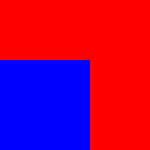``````pic1=picxproblem(5,5,"B:blue R:red",
columns="b5 b5 r5 r5 r5",
rows="r5 r5 b5 b5 b5")
success=pic1.solve(check_constraints=True) #You can uncomment the lines showing constraints violation to see it.
print(success)
pic1.topix()
``````
``````False
``````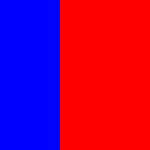``````pic2=picxproblem(5,5,"R:red Y:yellow",
columns="y5 R2Y3 r5 R1y4 y5",
rows="R1Y4 r2Y3 R1Y4 R1Y4 r3Y2")
pic2.solve(check_constraints=True)
pic2.topix()
``````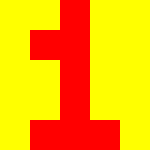``````pic3=picxproblem(5,5,"B:lightskyblue K:black M:chocolate",
columns="m4B1 m3b2 m2k2B1 m3b2 m4B1",
rows="b5 M3B2 m5 M4K1 M4K1")
pic3.solve()
pic3.topix()
````````````pic4=picxproblem(5,5,"P:mediumpurple B:black Y:yellow",
columns="B1P4 b3y2 b3y2 b3y2 B1P4",
rows="b3P2 b3P2 b5 y3P2 y3P2")
pic4.solve()
pic4.topix()
``````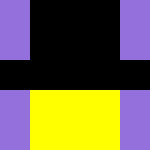``````pic4=picxproblem(5,5,"W:white B:black Y:yellow",
columns="W1Y3B1 Y3B2 y3B2 Y3B2 W1Y3B1",
rows="b5 y5 W2Y1B2 y5 Y4B1")
pic4.solve()
pic4.topix()
``````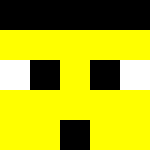The following problem asks for guess (at cell 1,4)

``````pic5=picxproblem(5,10,"B:blue G:green M:brown R:red",
columns="B1G6r3 b2G5r3 b2g2m6 b2G5r3 B1G6r3",
rows="B1G4 b3G2 B4M1 G4M1 G4M1 G4M1 G2M1R2 M1R4 G1R4 g3R2")
pic5.solve()
pic5
``````
 1 ② ② ② 1 6 5 ② 5 6 ⑥ ③ ③ ③ ③ 1 4 ③ 2 4 1 4 1 4 1 4 1 2 1 2 1 4 1 4 ③ 2
``````pic6=picxproblem(10,15,"M:brown G:slategray P:pink B:black",
columns="M1G12p2 M1G12p2 M5G5P1B4 M5g2p4b4 M5g2p4b4 M7g2p2B4 M6p4B5 M3G1P1B10 M2G6P3B4 G11p2B2",
rows="m4G5B1 M3G5b2 M2G5b3 m9B1 G3P3B4 G3P4B3 G4p6 G6p4 m4G5B1 m6G1p2B1 m7p2B1 g2p2b6 g3p2b5 G6B4 G5B5")
pic6.solve()
pic6.topix()
``````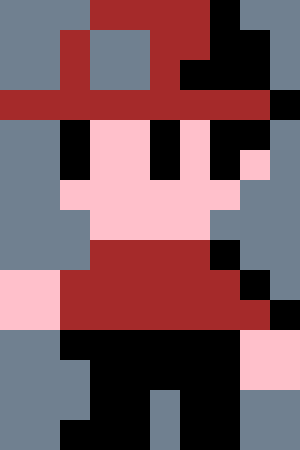``````pic7=picxproblem(10,15,"B:lightblue Y:yellow M:brown K:black",
columns="B4Y6M1K4 B3Y5M4K3 B2Y4M6k3 b2Y5M5k3 b6y7m2 b6y3m3k3 b7y2m2k4 b6y2m3k4 b6y2M7 b7y7M1",
rows="B9Y1 b6Y3M1 b6y4 B8Y1M1 B7y2M1 b7y2M1 B3Y1M6 Y1m9 Y6m4 b3y7 y6m2k2 Y3M4K3 Y2M3K5 Y2M1K7 Y2M1K7")
``````
``````pic7.solve(showprogress=False)
pic7
``````
 4 3 2 ② ⑥ ⑥ ⑦ ⑥ ⑥ ⑦ 4 3 ③ ③ ③ ④ ④ 1 4 6 5 ② ③ ② ③ 7 1 6 5 4 5 ⑦ ③ ② ② ② ⑦ 9 1 ⑥ 1 3 ⑥ ④ 8 1 1 7 1 ② ⑦ 1 ② 3 6 1 ⑨ 1 ④ 6 ③ ⑦ ② ② ⑥ 3 4 3 5 3 2 7 1 2 7 1 2

## Display an animation of the whole process

We will manually stitch all animations (some are longer than others) and display the result!

``````class gif():
def __init__(self, filename):
import base64
``````gif("week.gif")